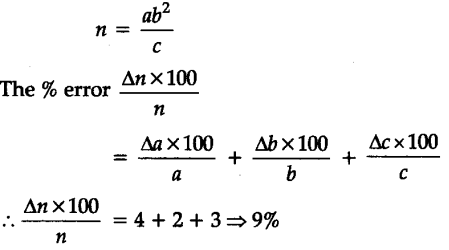# A physical quantity X is connected from X = a\${{b}^{2}}\$/C. Calculate percentage error in X, when % error in a, b, c are 4,2 and 3 respectively